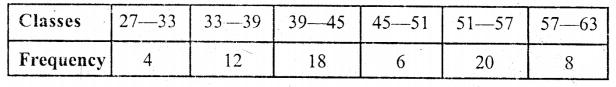## ML Aggarwal Class 9 Solutions for ICSE Maths Chapter 20 Statistics Chapter Test

Question 1.
Find the mean and the median of the following set of numbers :
8, 0, 5, 3, 2, 9, 1, 5, 4, 7, 2, 5
Arranging in descending order 0, 1, 2, 2, 3, 4, 5, 5, 5, 7, 8, 9
Here N = 12 which is evenQuestion 2.
Find the mean and the median of all the (positive) factors of 48.
Positive factors of 48 are 1, 2, 3, 4, 6, 8, 12, 16, 24, 48
which are 10 in numbers which is evenQuestion 3.
The mean weight of 60 students of a class is 52.75 kg. If the mean weight of 35 of them is 54 kg, find the mean weight of the remaining students.
Mean weight of 60 students = 52.75 kg
∴ Total weight of 60 students = 52.75 × 60 kg = 3165 kg
Mean weight of 35 students among them = 54 kg
∴ Total weight of 35 students = 54 × 35 kg = 1890 kg
∴ Remaining students = 60 – 35 = 25 and total weight of 25 students
= 3165 – 1890 = 1275 kg
∴ Mean weight of 25 students = 1275 ÷ 25 = 51 kg

Question 4.
The mean age of 18 students of a class is 14.5 years. Two more students of age 15 years and 16 years join the class. What is the new mean age ?
Mean age of 18 students = 14.5 years
∴ Then total age = 14.5 × 18 years = 261 years
Total age of 2 more students =15 + 16 = 31 years
and total age of 18 + 2 = 20 students = 261 + 31 = 292 years
∴ Mean age = $$\frac{292}{20}$$ = 14.6 years

Question 5.
If the mean of the five observations x + 1, x + 3, x + 5, 2x + 2, 3x + 3 is -14, find the mean of first three observations.
Mean of the 5 observations
x + 1, x + 3, x + 5, 2x + 2, 3x + 3 is 14Question 6.
The mean height of 36 students of a class is 150.5 cm. Later on, it was detected that the height of one student was wrongly copied as 165 cm instead of 156 cm. Find the correct mean height.
Mean height of 36 students of a class = 150.5 cm
Total height = 150.5 × 36 = 5418 cm
Difference in height which was wrongly copied = 165 + 56 = 9 cm
Actual height = 5418 – 9 = 5409 cm
∴ Actual mean height = $$\frac{5409}{36}$$
= 150.25 cm

Question 7.
The mean of 40 items is 35. Later on, it was discovered that two items were misread as 36 and 29 Instead of 63 and 22. Find the correct mean.
Mean of 40 items = 35
∴ Total of 40 items = 35 × 40 = 1400
Difference in two items which were wrongly read = (63 + 22) – (36 + 29)
= 85 – 65 = 20
∴ Actual total = 1400 + 20 = 1420
and correct mean = $$\frac{1420}{40}$$ = 35.5

Question 8.
The following observations have been arranged in ascending order. If the median of the data is 63, find the value of x : 29, 32, 48, 50, x, x + 2, 72, 75, 87, 91
Here number of data is 10 which is evenHence x = 62

Question 9.
Draw a histogram showing marks obtained by the students of a school in a Mathematics paper carrying 60 marks :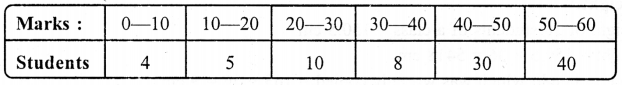We represent marks on x-axis and no. of students on y-axis and draw a histogram as given below:Question 10.
In a class of 60 students, the marks obtained in a surprise test were under: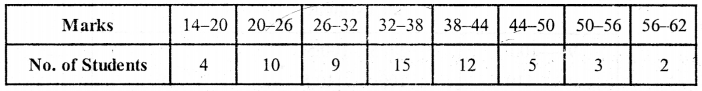Represent the above data by a histogram and a frequency polygon.
Solution:
We take marks on the x-axis and no. of students on the y-axis. Then, we draw a histogram by given data, Then by joining the mid-point of each class with .v-axis, we get a frequency polygon as shown.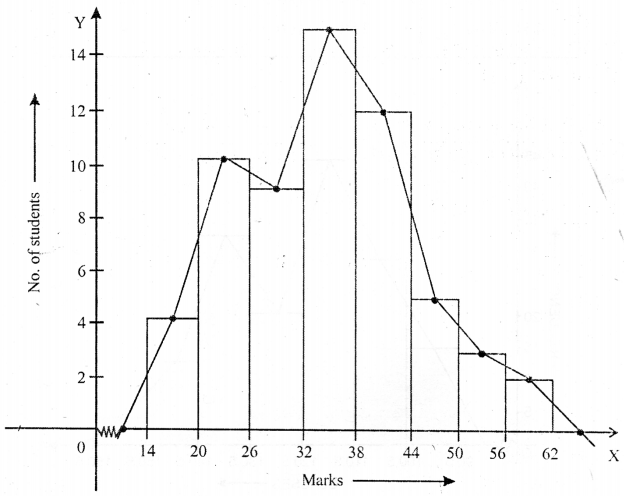Question 11.
Construct a combined histogram and frequency polygon for the following distribution :We represent classes on x-axis and frequency ony-axis and draw first histogram and there front it, we draw a frequency polygon as shown below : Firstly we write the classes in contineous frequency classes :Question 12.
The electricity bills (in rupees) of 40 houses in a locality are given below :Form a frequency distribution table with a class size of 10. Also represent the above data with a histogram and frequency polygon.
Least term = 52
Greatest term =129
Range = 129 – 52 = 77
Now making in a frequency disteribution table :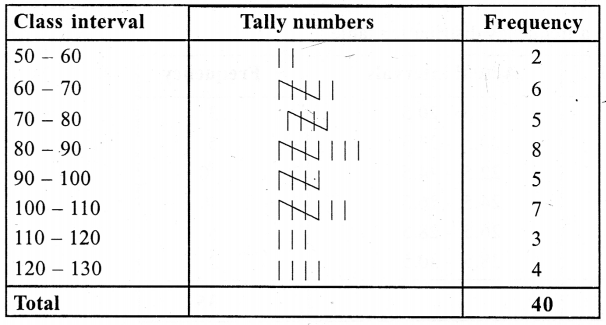Now taking class intervals on x-axis and frequency ony-axis, the histogram and frequency polygon is given below :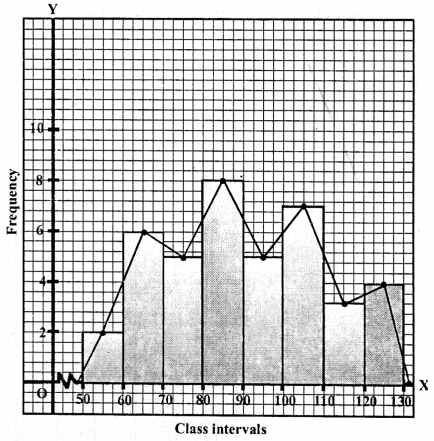Question 13.
The data given below represent the marks obtained by 35 students :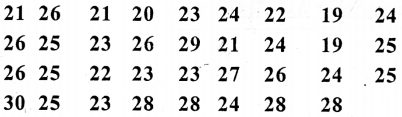Taking class interval 19 – 20, 21 – 22 etc., make a frequency distribution for the above data.
Construct a combined histogram and frequency polygon for the distribution.
Least mark = 19
Greatest marks = 30
Range = 30 – 19 = 11
Now we shall make a frequency distribution table :Now taking class interval on x-axis and frequency on the y-axis, the histogram and frequency polygon is given below.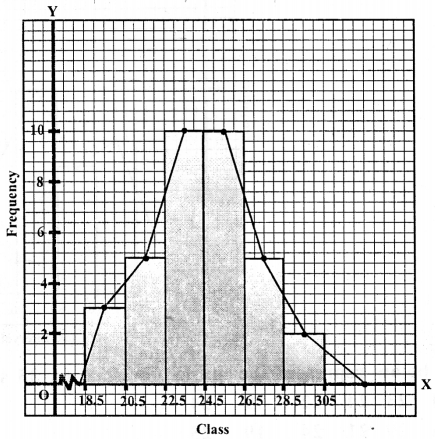Question 14.
The adjoining histogram and frequency polygon shows the ages of teachers in a school. Answer the following :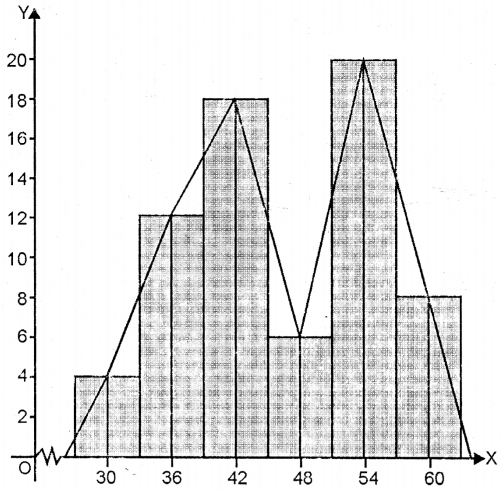(i) What is the class size of each class ?
(ii) What is the class whose class mark is 48 ?
(iii) What is the class whose frequency is maximum ?
(iv) Construct a frequency tabic for the given distribution.
(ii) Class size 45—51 lias class mark $$\frac{45+51}{2}=\frac{96}{2}$$ = 48